#### Thank you for registering.

One of our academic counsellors will contact you within 1 working day.

Please check your email for login details.

Click to Chat

1800-1023-196

+91-120-4616500

CART 0

• 0
MY CART (5)

Use Coupon: CART20 and get 20% off on all online Study Material

ITEM
DETAILS
MRP
DISCOUNT
FINAL PRICE
Total Price: Rs.

There are no items in this cart.
Continue Shopping• Complete JEE Main/Advanced Course and Test Series
• Offered Price: Rs. 15,900
• View Details

# Chapter 15: Areas Related To Circles Exercise – 15.3

### Question: 1

AB is a chord of a circle with center O and radius 4 cm. AB is of length 4 cm and divides the circle into two segments. Find the area of the minor segment.

### Solution:

Given data: Radius of the circle with center 'O', r = 4 cm = OA = OB

Length of the chord AB = 4 cm

OAB is an equilateral triangle and angle AOB = 60° + θ

Angle subtended at centre θ = 60°

Area of the segment (Shaded region) = (Area of sector) - (Area of triangle AOB)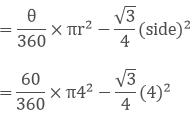On solving the above equation, we get, = 58.67 - 6.92 = 51.75 cm2

Therefore, the required area of the segment is 51.75 cm2

### Question: 2

A chord PQ of length 12 cm subtends an angle 120 at the center of a circle. Find the area of the minor segment cut off by the chord PQ.

### Solution:

We know that, Area of the segment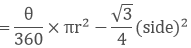We have, ∠POQ = 120 and PQ = 12cm

PL = PQ × (0.5) = 12 × 0.5 = 6 cm

Since, ∠POQ = 120

∠POL = ∠QOL = 60

In triangle OPQ, we have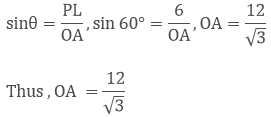Now using the value of r and angle θ. We will find the area of minor segment.### Question: 3

A chord of circle of radius 14 cm makes a right angle at the centre. Find the areas of minor and major segments of the circle.

### Solution:

Given data: Radius (r) = 14 cm

Angle subtended by the chord with the centre of the circle, θ = 90°

Area of minor segment (ANB) = (Area of ANB sector) - (Area of the triangle AOB)

= θ/360 × πr2 - 0.5 × OA × OB

= 90/360 × π142 - 0.5 × 14 × 14

= 154 - 98 = 56 cm2

Therefore the area of the minor segment (ANB) = 56 cm2

Area of the major segment (other than shaded) = area of circle - area of segment ANB = πr2 - 56 cm2

= 3.14 × 14 × 14 - 56

= 616 - 56

= 560 cm2

Therefore, the area of the major segment = 560 cm2.

### Question: 4

A chord 10 cm long is drawn in a circle whose radius is 52 cm. Find the area of both segments.

### Solution:

Given data: Radius of the circle, r = 5√2 cm = OA = OB

Length of the chord AB = 10 cm

In triangle OAB,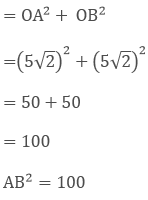Hence, Pythagoras theorem is satisfied.

Therefore OAB is a right angle triangle.

Angle subtended by the chord with the centre of the circle, θ = 90°

Area of segment (minor) = shaded region = area of sector - area of triangle

OAB = θ/360 × πr2 - 0.5 × OA × OB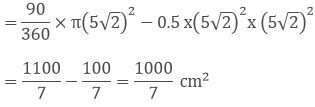Therefore, Area of segment (minor) = 1000/7 cm2.

### Question: 5

A chord AB of circle of radius 14 cm makes an angle of 60° at the centre. Find the area of the minor segment of the circle.

### Solution:

Given data: radius of the circle (r) = 14 cm = OA = OB

Angle subtended by the chord with the centre of the circle, θ = 60°

In triangle AOB, angle A = angle B [angle opposite to equal sides OA and OB] = x

By angle sum property, ∠A + ∠B + ∠O = 180

x + x + 60° = 180°

2X = 120°, x = 60°

All angles are 60°, triangle OAB is equilateral OA = OB = AB = area of the segment (shaded region in the figure) = area of sector area of triangleOn solving the above equation we get,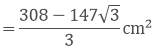Therefore, area of the segment (shaded region in the figure)### Question: 6

Ab is the diameter of a circle with centre 'O'. C is a point on the circumference such that ∠COB = θ. The area of the minor segment cut off by AC is equal to twice the area of sector BOC. Prove that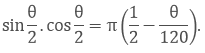### Solution:

Given data:  AB is a diameter of circle with centre O, Also, ∠COB = θ

= Angle subtended Area of sector BOC = θ/360 × πr2

Area of segment cut off by AC = (Area of sector) - (Area of triangle AOC)

∠AOC = 180 - θ ∠AOC and ∠BOC from linear pair] Area of sectorIn triangle AOC, drop a perpendicular AM, this bisects ∠AOC and side AC.

Now, In triangle AMO,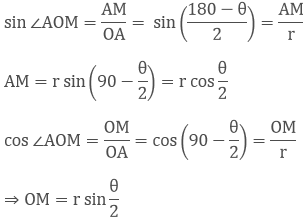Area of segmentArea of segment by AC = 2 (Area of sector BOC)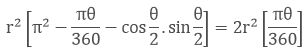On solving the above equation we get,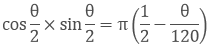Hence proved that,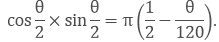### Question: 7

A chord a circle subtends an angle θ at the center of the circle. The area of the minor segment cut off by the chord is one-eighth of the area of the circle. Prove that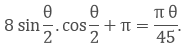### Solution:

Let the area of the given circle be = r

We know that, area of a circle = πr2

AB is a chord, OA and OB are joined. Drop a OM such that it is perpendicular to AB, this OM bisects AB as well as

∠AOM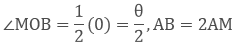Area of segment cut off by AB = (area of sector) - (area of the triangle formed)On solving the above equation we get,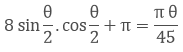Hence proved,## Complete JEE Main/Advanced Course and Test Series

OFFERED PRICE: Rs. 15,900
View Details Xpress Buy

### Course Features

• 731 Video Lectures
• Revision Notes
• Test paper with Video Solution
• Mind Map
• Study Planner
• NCERT Solutions
• Discussion Forum
• Previous Year Papers

### Course Features

• 728 Video Lectures
• Revision Notes
• Previous Year Papers
• Mind Map
• Study Planner
• NCERT Solutions
• Discussion Forum
• Test paper with Video Solution